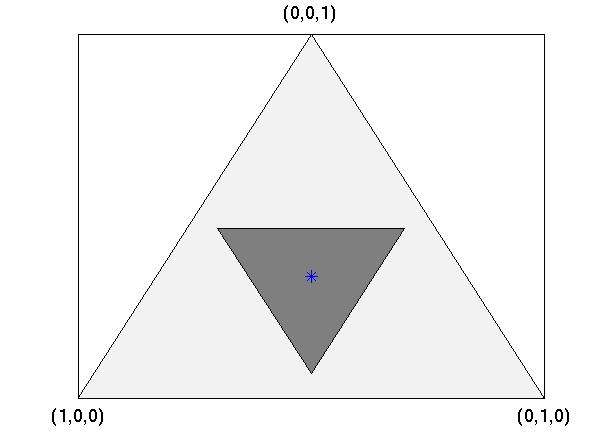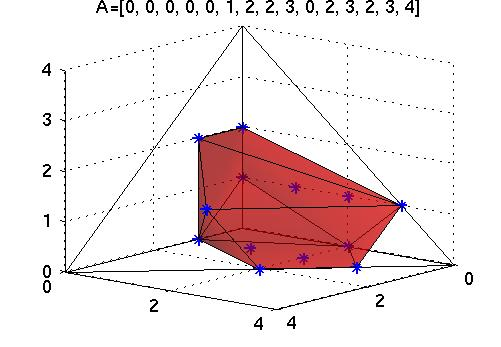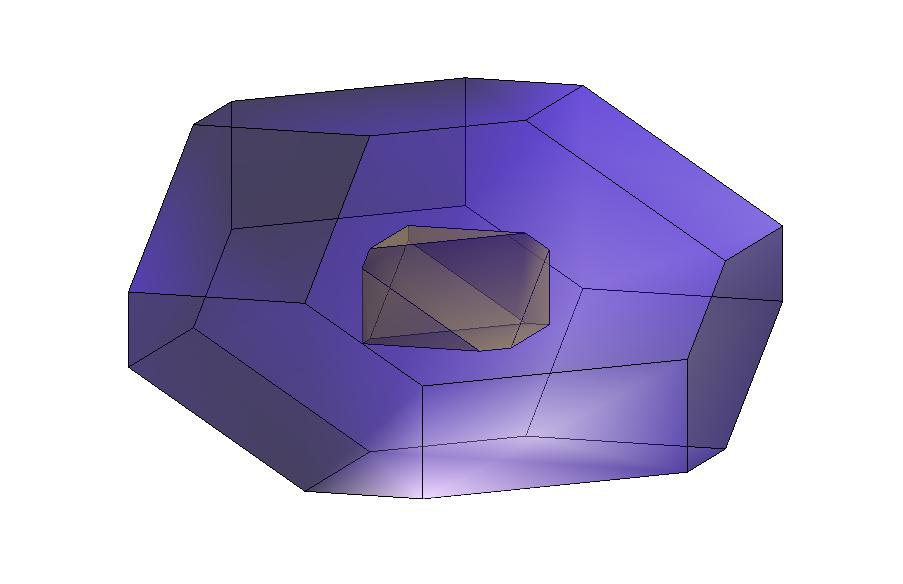# TUGlab

## Transferable Utility Matlab Toolbox#### Authors

• Miguel Ángel Mirás CalvoDepartment of Mathematics. University of Vigo
• Estela Sánchez Rodríguez. Department of Statistics and Operations Research. University of Vigo

We wish to acknowledge the support of the Santiago Game Theory Group.

##### Textbook (in Spanish)

TUGlab (Transferable Utility Games laboratory) is a Matlab set of scripts that could serve as a helpful complement to the books and other materials used in introductory courses on cooperative game theory. Its main goal is to emphasize the geometrical aspects of cooperative game theory. TUGlab offers to both the instructor and the student a tool to compute and visualize basic concepts for any given 3 or 4 players coalitional game. It allows the user to experiment at will with games without worrying about the mathematical complexity of the computations. That is the power of this platform: its direct and flexible way of going to the heart of the concepts overcoming the mathematical complexity.

The TUGlab toolbox works on any implementation of the later releases of Matlab on Unix, PC or Macintosh. It is a collection of 58 files including:

1. The main scripts (29 M-files) defining the procedures concerning game theory concepts.
2. Auxiliary scripts (27 M-files) necessary for the computations but not directly related to game theory.
3. Data files (2 MAT-files)

To install it, just copy and paste the folder TUGlab on your hard disk. Then, include this directory in the Matlab search path. All the TUGlab functions can be run directly from the Matlab Command Window. The characteristic function of the game must be introduced as a vector
A=[v(1) v(2) v(3) v(12) v(13) v(23) v(123)], for 3-person games, or
A=[v(1) v(2) v(3) v(4) v(12) v(13) v(14) v(23) v(24) v(34) v(123) v(124) v(134) v(234) v(1234)], for 4-person games.

Please, acknowledge the authors if you make use of TUGlab in your teaching or research.

#### Software and documentation

The 58 Matlab M-files that are part of the TUGlab toolbox. (TUGlab.zip)

The folder Tutorials.zip contains:

• examples.m: A list of selected examples of interesting coalitional games.
• parametric3.m: A Matlab script that draws the cores of the parametric family of 3-player games given by A(p)=[0 0 0 p p p 1], p=0,…,2/3. If p<=1/2 the game A(p) is convex. For p>1/2 the game A(p) is not convex and, therfore, the core and the Weber set do not coincide. For p=8/15, the core and the Weber set are superimposed. As p approaches 2/3, the core shrinks to a point.
• parametric4.m: A Matlab script that draws the cores of the parametric family of 4-persons games given by A(p)=[0 0 0 0 c(p) c(p) c(p) c(p) c(p) c(p) p p p p 22], with c(p)=max(0,p-5) and p=0,…,14. If p<=10 the game A(p) is convex. For p>10 the game A(p) is not convex and, therfore, the core and the Weber set do not coincide. For p=12, we get an example proposed by Derks, 2001: the core has 24 extreme points none of which is a marginal vector.For this particular value p=12, we draw the Weber set, the core and the marginal vectors.
• moviesource.m and simulation.mA Matlab movie to compare the behavior of the Shapley value, the nucleolus and the corecenter. The «mouviesource.m» file creates the movie and stores it in the file «simulation.mat». Once you have created the movie, if you want to see it again run the script «simulation.m». The frames show the core of the parametric game A(p)=[0,0,0,p,5,5,10], p=0,…,10, and compare the Shapley value, the corecenter and the nucleolus. The game is convex in the parameter range [0,5]. The nucleolus, N, the corecenter, CC, and the Shapley value, Sh, coincide for p=0. The nucleolus remains unchanged, N=(2.5,2.5,5), if the parameter p belongs to [0,2.5] while CC and Sh varied. The three values converge to (10/3,10/3,10/3) when the parameter p moves from 2.5 to 5. The game is not convex in the parameter range [5,10], the core is a triangle that shrinks to the point (5,5,0) as p approaches 10, so CC and N coincide and converge to that point. But, the Weber set grows so Sh moves away from the other two values.